## Lifter theory

Force/Power optimization
by Evgenij Barsoukov

Courtesy of Evgenij Barsoukov
Created on April 30, 2002 - JLN Labs - Updated on April 30, 2002

The original document can be found at : http://sudy_zhenja.tripod.com/lifter_theory/Force.htm

Force/Power optimization

Using derived above equations for current/thrust and current/voltage relation it is possible to tackle the main problem - how to improve thrust/power ration of a lifter to make it a more attractive flying device compared to conventional once. How would thrust/power ratio change if we adjust voltage, distance wire/collector, and wire radius? Results are shown below.

Used equations (see derivation at lftheory.htm#eqn ):

Thrust/current :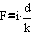Current/voltage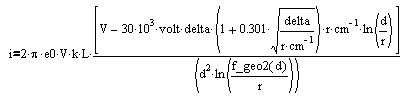Here :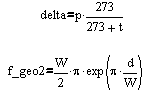And values of parameter used in calculation are :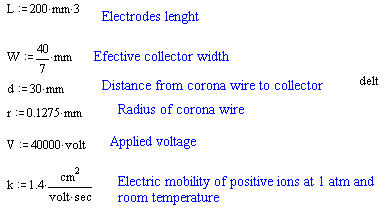1.   Voltage optimization

Voltage was calculated for 100 points from minimal corona initiation voltage (6.9kV) calculated by Peek's equation for given distance, until voltage 100kV.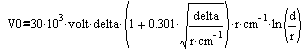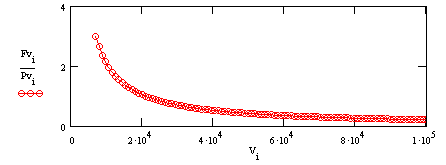Note that spark discharge might occur before 100kV.

Here is resulting Thrust (in gram)/Power (in W) relation

..and Thrust/Voltage relation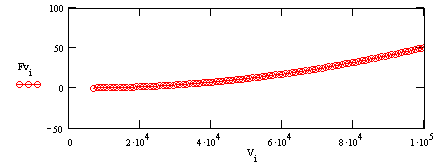As you could expect, thrust is increasing with voltage, however the efficiency (thrust/power) is decreasing rapidly from maximal 3 g/W to very small values.

2.    Wire/collector distance optimization

Thrust is calculated by changing d from 30mm to 1m

Resulting Thrust/Power ration is shown below :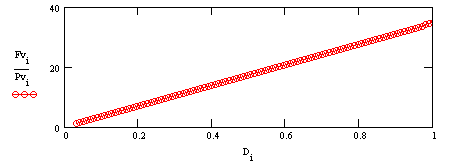Exciting result is that thrust/power relation is increasing proportionally with distance and can reach huge values as 40 gm/W! However, absolute thrust is rapidly decreasing with distance as can be seen below :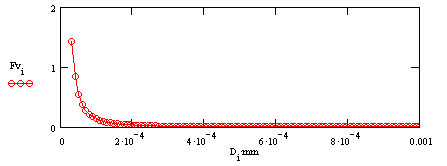Nevertheless, absolute thrust can be increased by increasing the length of wire/collector. Therefore increasing distance gives clear way of almost unrestricted efficiency increase.

Radius was changed from gauge 50 (0.015 mm) to gauge 20 (1.5mm). Resulting thrust/power relation is given below :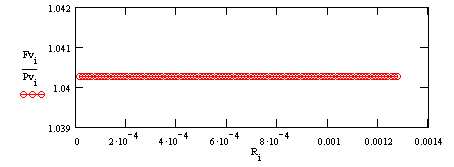It is great to see, that efficiency does not change, but thrust does! See below absolute thrust/radius dependence :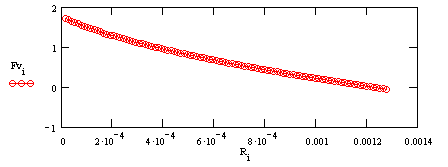With decreasing radius thrust increases a lot, without losing efficiency!

### Conclusion

• Increasing the wire/collector distance by simultaneous increase of wire/collector lenght can allow to achieve thrust/power ratios up to 40g/W.